### Home > PC > Chapter 1 > Lesson 1.4.2 > Problem1-151

1-151.
1. State the parent graph, the transformation and the equation for each graph below. Homework Help ✎

1.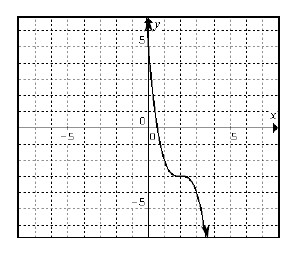2.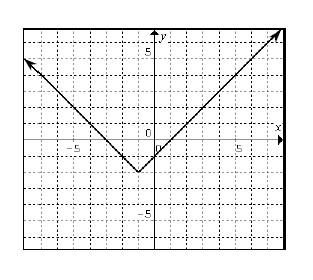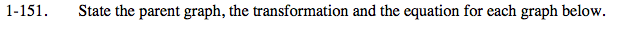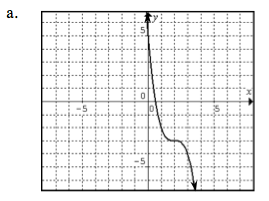$\textit{y} = \textit{x}^3$

1. Flip over y-axis → y = −x³
2. Shifted right two units → y = −(x −2)³
3. Shifted down three units → y = −(x −2)³ −3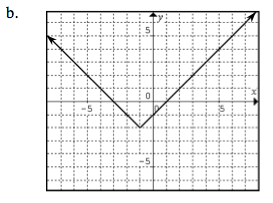$y = |x|$

$\text{Shifted left one unit}\Rightarrow y =\left | x+1 \right |$

$\text{Shifted down two units}\Rightarrow y =\left | x+1 \right |-2$